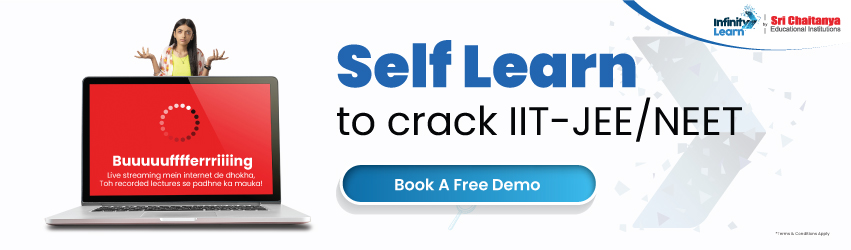NCERT Solutions for Class 12 Maths Chapter 9 Differential Equations (Ex 9.6) Exercise 9.6

# NCERT Solutions for Class 12 Maths Chapter 9 Differential Equations (Ex 9.6) Exercise 9.6infinitylearn surge self learn
infinitylearn starter package

All of the questions that must be studied are answered in the NCERT Solutions for Class 12 Maths Chapter 9 Differential Equations Exercise 9.6. With the appropriate notes, NCERT Maths Class 12 Exercise 9.6 Solutions are straightforward to understand. The Class 12 Maths Chapter 9 Exercise 9.6 Solutions from Infinity Learn are quite useful. Infinity Learn has developed notes that are simple to comprehend for students who desire to excel in their exams. Download the CBSE Class 12 Maths Chapter 9 Differential Equations PDF for free.

Do you need help with your Homework? Are you preparing for Exams? Study without Internet (Offline)
×
Exercise 9.6

Need FREE NCERT/CBSE/IIT-JEE/NEET Study Material?

NCERT Solutions for Class 12 Maths Chapter 9 Differential Equations Exercise 9.6

When it comes to exam preparation, the NCERT solutions for Ex 9.6 Class 12 Maths are considered to be the finest alternative for CBSE students. There are a lot of exercises in this chapter. We’ve included the Exercise 9.6 Class 12 Maths NCERT solutions in PDF format on this page. You can either download this solution or study it immediately from our website/app.

Infinity Learn’s in-house subject matter specialists addressed the exercise’s problems/questions with great care and precision, adhering to all CBSE criteria. Any student in Class 12 who understands all of the concepts from the Maths textbook and is well-versed in all of the problems from the exercises can easily achieve the greatest possible grade on the final exam. Students can easily grasp the pattern of questions that may be asked in the exam from this chapter and also study the marks weightage of the chapter with the assistance of this Class 12 Maths Chapter 9 Exercise 9.6 solutions. So that they can adequately prepare for the final examination.

Apart from these NCERT solutions for Class 12 Maths Chapter 9 Exercise 9.6, this chapter contains numerous exercises with numerous questions. As previously said, our in-house subject specialists solve/answer all of these questions. As a result, all of these are guaranteed to be of high quality, and anyone can use them to study for exams. It is critical to grasp all of the topics in the textbooks and solve the questions from the exercises supplied next to them in order to achieve the highest possible grade in the class. Don’t put it off any longer. For better exam preparation, get the NCERT solutions for Class 12 Maths Chapter 9 Exercise 9.6 from the Infinity Learn website today. If you have the Infinity Learn app installed on your phone, you may also download it from there. The nice thing about these solutions is that they can be used both online and offline.

FAQ:

1. Are there answers to all of the textbook problems in the NCERT Solutions for Class 12 Maths Chapter 9?

Ans. The NCERT Solutions for Class 12 Maths Chapter 9 are created by subject specialists and are accessible in PDF format. These solutions are based entirely on the most recent CBSE Syllabus 2021-22, and they cover all of the key ideas for the second-term exam. The textbook questions are addressed in a step-by-step fashion based on the weighting of the marks in the second-term exams. Infinity Learn’S has chapter-by-chapter and exercise-by-exercise PDF links that students can use to get their questions answered quickly.

1. Is Chapter 9 of Class 12 Maths difficult?

Ans. Math becomes easier for each student with practice. Maths will be simple for that kid after he or she has a firm grasp of the fundamental principles and has learned the techniques to solve each question. As a result, it’s critical to master the fundamentals and practice thoroughly for all types of queries. In terms of complexity, the Class 12 Maths syllabus contains a combination of questions. The solutions may be found on the NCERT Solutions Class 12 Maths Chapter 9 website.

1. What is the finest NCERT Class 12 Maths Chapter 9 Solution book?

Ans. By going to the Infinity Learn website and looking for Class 12 Maths solutions, you may get NCERT Class 12 Maths Solutions. Aside from that, you’ll get access to a number of courses that will help you get good grades on math exams. The solutions to the exercises may be found on the NCERT Solutions Class 12 Maths Chapter 9 page. To download a PDF of the solutions, click on it.

Join Infinity Learn Regular Class Programme!